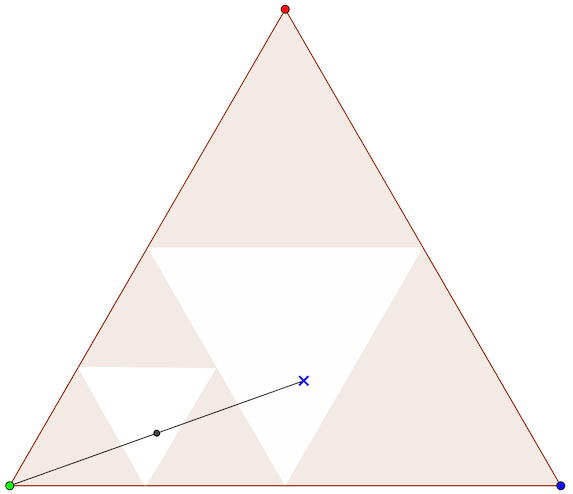Fractals Part III

This is the last of three activities that explore the construction and visualisation of fractals.

Although arising from simple processes, fractals exhibit infinite complexity, and exist at the nexus of mathematics, nature, and art. No matter how closely you look at a fractal, however much you zoom in, they remain equally complex (i.e. bumpy). They are nowhere smooth!

Two key concepts are:

• Recursion – i.e. repeating a transformation on its own output. This may remind you of Russian dolls, or the infinite reflections in parallel mirrors, or even the process of revising an essay.
• Self similarity – the features of the whole object are repeated infinitely often in its parts, at finer and finer scales. At all magnifications, the object exhibits the same complex structure.

In this activity we use repeated transformations of individual points to generate fractals. The first of these employs the simple Chaos Game with quite a remarkable output. Slightly more complicated transforms generate the Barnsley Fern, and, perhaps the most famous fractal of all, the Mandelbrot Set.

The Chaos Game
Building fractals point by point
Welcome to the Chaos Game. The game is played with three coloured points in a triangle as below, and a single starting point somewhere in the middle. (It doesn't actually matter exactly where.) To play, click the spinner to select a random colour, and then colour the point exactly half way between the starting point and the vertex of the chosen colour. This midpoint becomes the new starting point, and the process is repeated.

Constructing and colouring points in this way gradually makes a pattern inside the triangle. Can you guess what this pattern will be?

It takes many points to see the pattern clearly. Use the buttons below to spin faster, and eventually avoid spinning entirely and just get the computer to do everything for you. Many interest variations are possible by changing the number of points and the way of choosing the new point. The activity supports versions that use a triangle, a square and a hexagon.

Click here for an explanation of the shape produced by playing the Chaos Game.

Let's start by thinking about why there are no points in the middle triangle. As the diagram below suggests, for a line from one of the vertices to have a midpoint in the middle triangle, it would need to start from outside the original triangle. But our point is always inside the original triangle, and so we never generate any points in the middle.This explains why the middle triangle remains empty, but what about the other triangles? The reasoning is similar. For any of the smaller empty triangles, the starting point would have to be in one of the larger empty triangles as shown in the following diagram.This is an example of recursive reasoning, and so it perhaps not quite so surprising to have a fractal pattern result from the moves in the Chaos Game after all.

The resulting pattern approaches the Sierpiński gasket that we saw in the first fractal activity – Sierpiński Stamping.

Hide the explanation.

Barnsley's Fern

A crucial aspect of the Chaos Game is the random choice of vertex. Barnsley's Fern is another example of a random point iteration that produces a fractal pattern. The rules take any point (x,y) and return a new x and y according to:

1% of the time:x → 0,
y → 0.16 y
85% of the time:x → 0.85 x + 0.04 y,
y → −0.04 x + 0.85 y + 1.6
7% of the time:x → 0.2 x − 0.26 y,
y → 0.23 x + 0.22 y + 1.6
7% of the time:x → −0.15 x + 0.28 y,
y → 0.26 x + 0.24 y + 0.44

Click the Show button below to see this process in action.

Notice how each frond is a copy of the whole leaf – self similarity again.

Mandelbrot Set Explorer

The most famous fractal of all is undoubtedly the Mandelbrot Set. This is the set of points (c,d) for which the following iterative process converges.

x → x2 - y2 + c,
y → 2xy + d

The points are coloured black if the iteration converges, and thus are part of the set, but it is at the boundary of the set that things get most interesting. The boundary curve is infinitely complicated – like the Heighway Dragon, it is a curve with fractal dimension 2. When the iteration doesn't converge, a colour is chosen based on how rapidly the iteration diverged. This creates some wonderful patterns.

Another way to visualise the dynamics is to fix on a particular point (c,d), and plot how sensitive the iteration is to different starting points. The shape that results is known as a Julia Set. For points near the boundary of the Mandelbrot set, the Julia Set itself exhibits incredibly beautiful, fractal behaviour.

The app below allows you to explore the Mandelbrot set – click and drag to zoom in on a particular area, or drag backwards to restore the previous zoom. One interesting activity is to search for smaller copies of the shape, then zoom in on them and find copies of the copies etc. The Palette button cycles through different ways to colour the fractal.

To see the Julia set corresponding to a particular point select the Julia Set button, then click and drag until the crosshairs are at your point of interested, then release. The corresponding Julia set will be shown, and you can explore in the same way as for the original Mandelbrot display.

Hitting Reset will restore the Mandelbrot set to the display.